# Lecture 11 — Decisions Part 2¶

## Overview — Logic and Decision Making, part 2¶

• Program structure
• Debugging and “hardening” a simple function
• A bit of design and a bit of a random walk
• More on logic - the key to getting programs right
• Boolean logic
• Nested if statements
• Assigning boolean variables

## Part 1: Program Structure¶

Programming requires four basic skills:

1. Develop a solution.

• Start with small steps, solve them, and then add complexity
2. Structure your code.

• Move repeated code into functions

• Make your code easy to read and modify. Use meaningful variable names and break complex operations into smaller parts.

• Place values in variables so they are easy to change.

• Include comments for important functions, but do not clutter your code with unnecessary comments. A classic example of a completely unnecessary comment is

x += 1   # increment x

• Document assumptions about what is passed to a function.

• If your function is meant to return a value, make sure it always does.

3. Test your code.

• Find all possible cases that your program should work for. As programs get larger, this is increasing hard.
• If you cannot check for all inputs, then you must then check your code for meaningful inputs: regular (expected inputs) and edge cases (inputs that can break your code).
4. Debug your code.

• Never assume an untested part of your code is bug free.
• Learn syntax well so that you can spot and fix syntax errors fast.
• Semantic errors are much harder to find and fix. You need strategies to isolate where the error is.
• Print output before and after crucial steps.
• Look at where your program crashed.
• Fix the first error first, not the biggest error. The first error may be the cause of bigger errors later in your code.
• Use a debugger.
• Simplify the problem: remove (by commenting them out) parts of your code until you no longer have an error. Look at the last code removed for a source of at least part of your errors.
• Test parts of your code separately and once you are convinced they are bug free, concentrate on other parts.

## Help with debugging¶

• Consider the following code to add the first n integers:

n = int(raw_input("Enter a positive integer ==> "))
sum = 0
i = 0
while i != n:
sum += i
i += 1
print 'Sum is', sum

• Does it work? For all inputs? Might it run forever? (We’ll ignore the fact that a for loop would be better here.)

• How might we find such an error?

• Careful reading of the code
• Insert print statements
• Use the Wing IDE debugger.
• We will practice with the Wing IDE debugger in class, using it to understand the behavior of the program. We will explain the following picture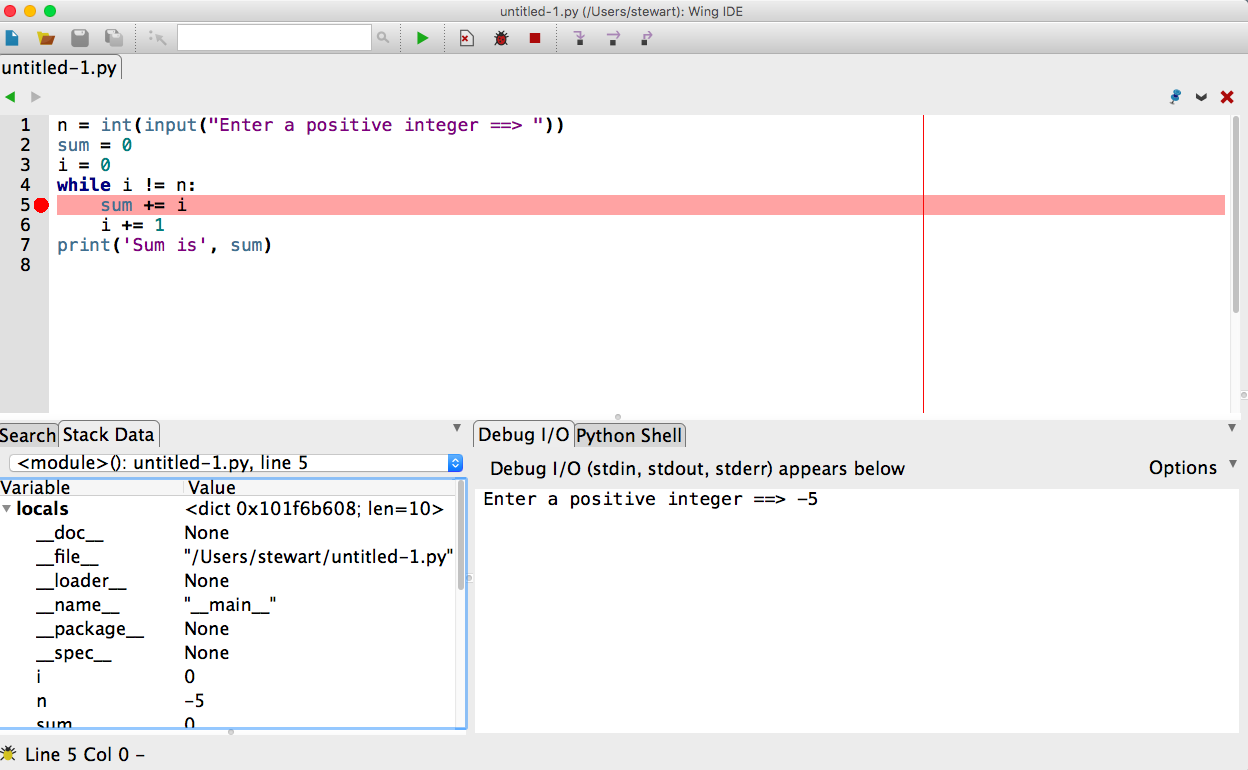and note the use of

• The hand, bug and stop symbols on the top of the display, and
• The Debug I/O and Stack Data at the bottom of the display.
• Debugging becomes crucial in tracking logic errors as well.

## Program organization¶

• Envision your code as having two main parts: the main body and the functions that help the main code.

• Make sure your functions do only one thing, so they are easy to test and may be used in many places.

• As we will see in an example below, in Python it is good practice to separate the functions and the main body with the following addition to the program structure:

if __name__ == "__main__"
# Put the main body of the program below this line

• This will have no apparent effect when a program is run. However, if a program is imported as a module into another program (like the utility code I have been giving you), any code within the above if block is skipped!

• This allows programs to work both as modules and stand alone code.

• When the primary purpose of your code is to provide functionality as a module, it is best to use the code in the main body to test the module functions.

## Example: Random Walk¶

• Many numerical simulations, including many video games, involve random events.

• Python includes a module to generate numbers at random. For example,

import random

# Print three numbers randomly generated between 0 and 1.
print random.random()
print random.random()
print random.random()

# Print a random integer in the range 0..5
print random.randint(0,5)
print random.randint(0,5)
print random.randint(0,5)

• We’d like to use this to simulate a “random walk”:

• Hypothetically, a (very drunk) person takes a step to the left or a step to the right, completely at random (equally likely to go left or right). This can be repeated over and over again until some stopping point is reached.
• If the person is on a platform with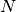steps and the person starts in the middle, this process is repeated until s/he falls off (reaches step 0 or step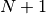)
• How many steps does this take?
• Many variations on this problem appear in physical simulations.

• We can simulate a step in two ways:

1. If random.random() returns a value less than 0.5 step to the left; otherwise step to the right.
2. If random.randint(0,1) returns 0 then step left; otherwise, step right.
• So, in summary, we want to start a random walk at position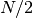and repeatedly take a step to the left or to the right based on the output of the random number generator until the walker falls off.

• We will solve this problem together during lecture. We we start by enumerating some of the needed steps and then solving them individually before putting together the whole program.

• Once we see the result we can think of several ways to change things and explore new questions and ideas. Remember, a program is never done!

## Review: Boolean Logic¶

• Invented / discovered by George Boole in the 1840’s to reduce classical logic to an algebra
• This was a crucial mathematical step on the road to computation and computers
• Values (in Python) are True and False
• Operators
• Comparisons: <, >, <=, >=, == !=
• Logic: and, or, not

## Truth Tables¶

• Aside to recall the syntax: and,or,not are lower case!

• If we have two boolean expressions, which we will refer to as ex1 and ex2, and if we combine their “truth” values using and we have the following “truth table” to describe the result

ex1 ex2 ex1 and ex2
False False False
False True False
True False False
True True True
• If we combine the two expressions using or, we have

ex1 ex2 ex1 or ex2
False False False
False True True
True False True
True True True
• Finally, using not we have

ex1 not ex1
False True
True False

## DeMorgan’s Laws Relating and, or, not¶

• Using ex1 and ex2 once again to represent boolean expressions, we have

not (ex1 and ex2) == (not ex1) or (not ex2)

• And,

not (ex1 or ex2) == (not ex1) and (not ex2)

• Also, distribution laws

ex1 and (ex2 or ex3) == (ex1 and ex2) or (ex1 and ex3)
ex1 or (ex2 and ex3) == (ex1 or ex2) and (ex1 or ex3)

• We can prove these using truth tables.

## Why Do We Care?¶

• When we’ve written logical expressions into our programs, it no longer matters what we intended; it matters what the logic actually does.
• For complicated boolean expressions, we may need to almost prove that they are correct
• We will work through the example of writing a boolean expression to decide whether or not two rectangles with sides parallel to each other and to the x and y axes intersect.

## Short-Circuited Boolean Expressions¶

• Python only evaluates expressions as far as needed to make a decision.

• Therefore, in boolean expression of the form

ex1 and ex2


ex2 will not be evaluated if ex1 evaluates to False. Think about why.

• Also, in boolean expression of the form

ex1 or ex2


ex2 will not be evaluated if ex1 evalues to True.

• This “short-circuiting” is common across many programming languages.

## Exercises¶

1. Write a boolean expression to determine if two circles, with centers at locations x0, y0 and x1, y1 and radii r0 and r1 intersect.

2. Suppose you have a tuple that stores the semester and year a course was taken, as in

when = ('Spring',2013)


Write a function that takes two such lists as arguments and returns True if the first list represents an earlier semester and False otherwise. The possible semesters are 'Spring' and 'Fall'.

## More Complicated/Nested If Statements¶

• We can place if statements inside of other if statements.

• To illustrate

if ex1:
if ex2:
blockA
elif ex3:
blockB
elif ex4:
blockD
else:
blockE

• We can also work back and forth between this structure and a single level if, elif, elif, else structure.

• We will work through this example in class.

## Example: Ordering Three Values¶

• Suppose three siblings, Dale, Erin and Sam, have heights stored in the variables hd, he and hs, respectively .
• We’d like code to print the names in order of height from greatest to least.
• We’ll consider doing this with nested if statements and with a single-level if, elif structure.

## Part 2 Exercise¶

1. In the following code, for what values of x and y does the code print 1, for what values does the code print 2, and for what values does the code print nothing at all?

if x>5 and y<10:
if x<5 or y>2:
if y>3 or z<3:
print 1
else:
print 2


The moral of the story is that you should be careful to ensure that your logic and if structures cover the entire range of possibilities!

2. Check that a string contains a float.

3. Suppose we represent the date as three integers in a tuple giving the month, the day and the year, as in

d = (2, 26, 2103)


Write a Python function called is_earlier that takes two date lists and returns True if the first date, d1 is earlier than the second, d2. It should return False otherwise. Try to write this in three different ways:

1. Nested ifs,
2. if - elif - elif - else,
3. A single boolean expression.

## Part 3: Storing Conditionals¶

• Sometimes we store the result of boolean expressions in a variable for later use:

f = float(raw_input("Enter a Fahrenheit temperature: "))
is_below_freezing = f < 32.0
if is_below_freezing:
print "Brrr.  It is cold"

• We use this to

• Make code clearer
• Avoid repeating tests

## Example from the Textbook¶

• Doctors often suggest a patient’s risk of heart disease in terms of a combination of the BMI (body mask index) and age using the following table:

Age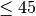Age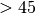BMI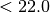Low Medium
BMI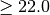Medium High
• Assuming the values for a patient are stored in variables age and bmi, we can write the following code

slim = bmi < 22.0
young = age <= 45

if young:
if slim:
risk = 'low'
else
risk = 'medium'
else:
if slim:
risk = 'medium'
else:
risk = 'high'


## Exercises¶

1. Rewrite the previous code without the nested if’s. There are several good solutions.
2. Make the code for checking whether two circles intersect unbreakable.
3. Write code to check if two rectangles intersect.

## Summary of Discussion of If Statements and Logic¶

• Logic is a crucial component of every program.
• Basic rules of logic, including DeMorgan’s laws, help us to write and understand boolean expressions.
• It sometimes requires careful, precise thinking, even at the level of a proof, to ensure logical expressions and if statement structures are correct.
• Many bugs in supposedly-working programs are caused by conditions that the programmers did not fully consider.
• If statements can be structured in many ways, sometimes nested several levels deep.
• Nesting deeply can lead to confusing code, however.
• Warning specifically for Python: you can easily change the meaning of your program by accidentally changing indentation. It is very hard to debug these changes.
• Using variables to store boolean values can make code easier to understand and avoids repeated tests.
• Make sure your logic and resulting expressions cover the universe of possibilities!